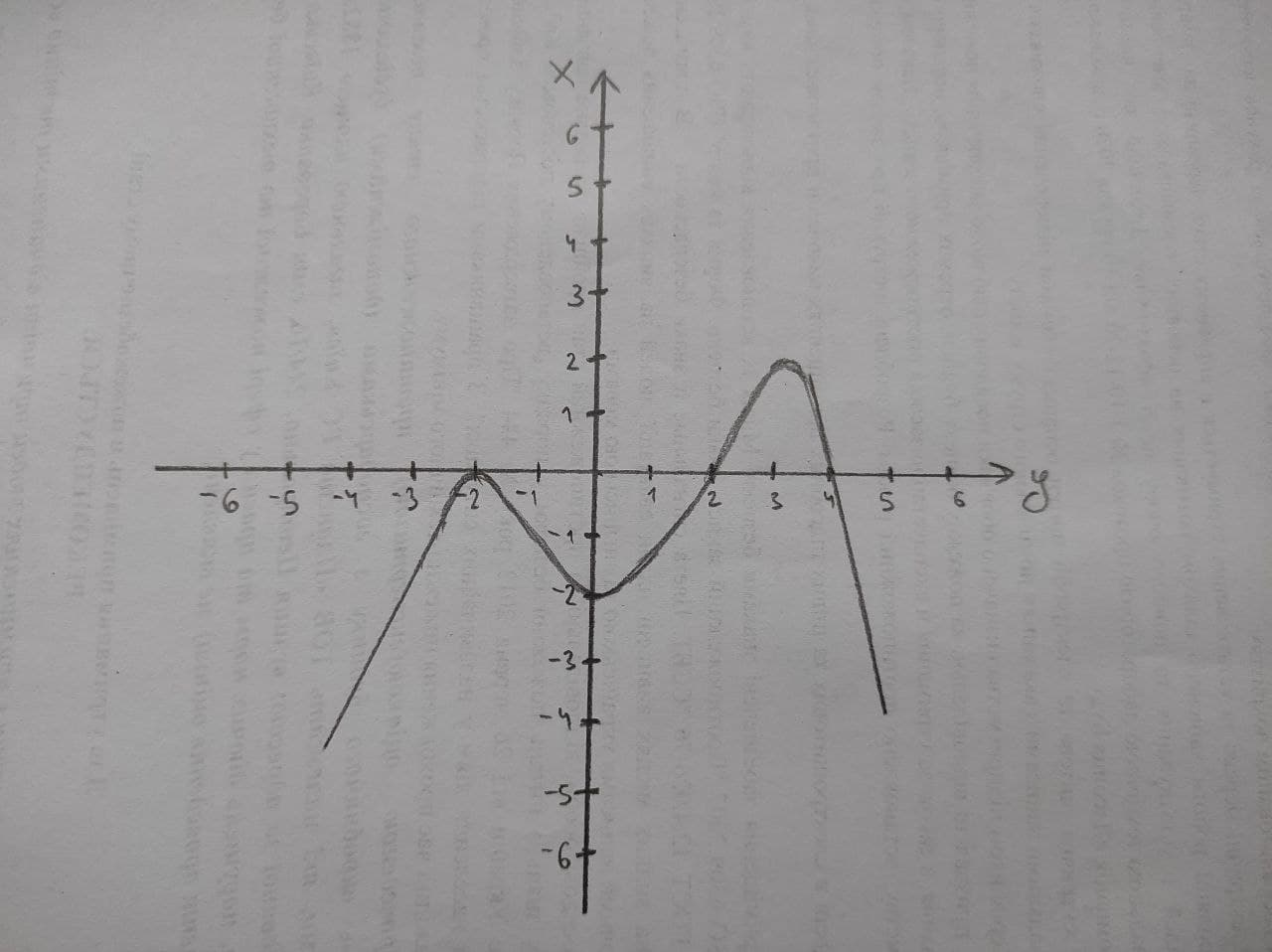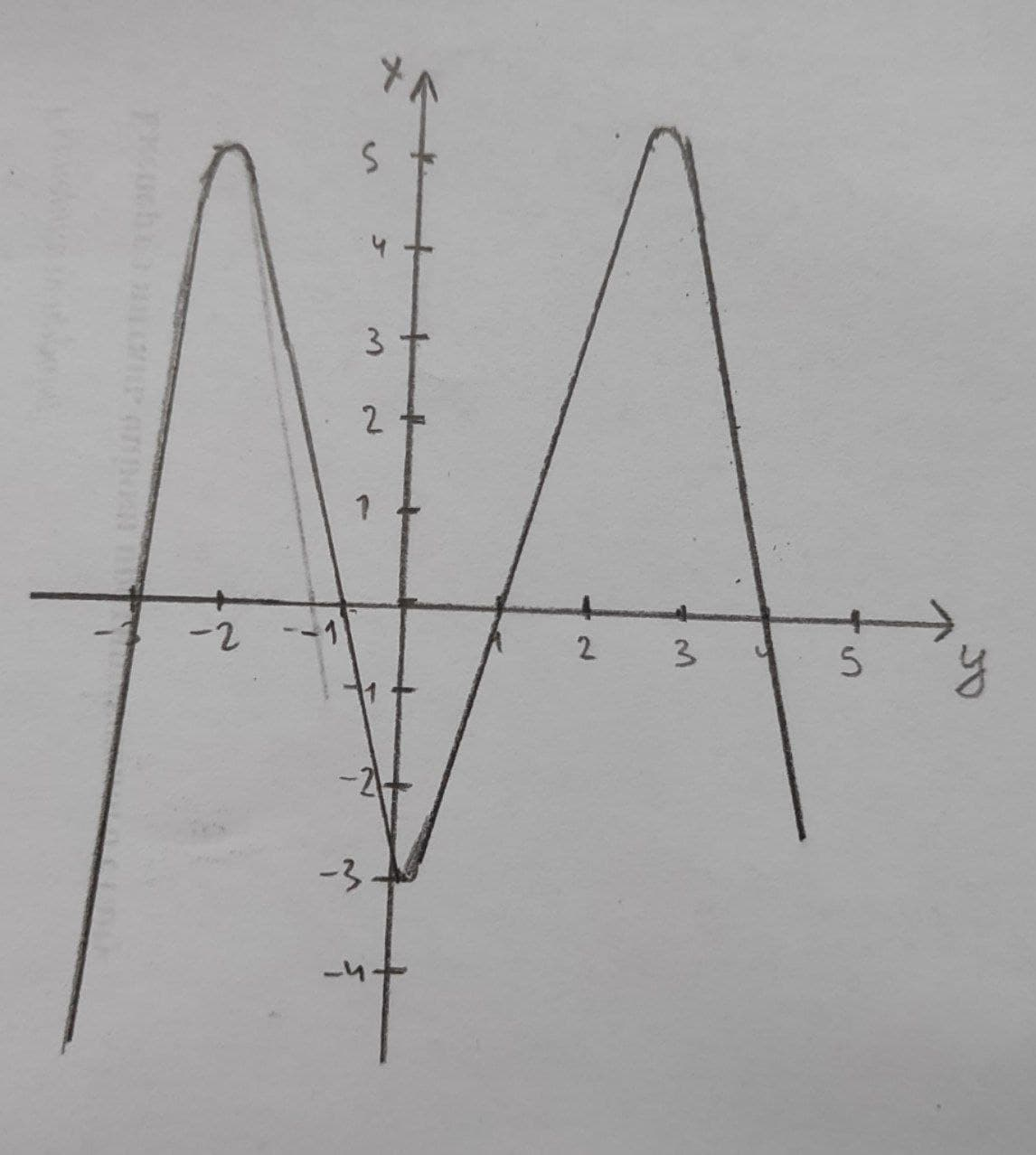# Algebra II: Polynomial graphs questions and answers

Recent questions in Polynomial graphsiohanetc 2021-03-08 Answered

### For the following exercises, use the given information about the polynomial graph to write the equation. Double zero at $$\displaystyle{x}=−{3}$$ and triple zero $$\displaystyle{a}{t}{x}={0}$$. Passes through the point (1, 32).foass77W 2021-03-07 Answered

### Write an equation for the polynomial graph:$$y(x)=?$$Haven 2021-02-25 Answered

### Write an equation for the polynomial graph:y(x)=?slaggingV 2021-02-25 Answered

### Graph the polynomial function. $$\displaystyle{f{{\left({x}\right)}}}=−{x}^{{{4}}}+{3}{x}^{{{3}}}−{x}+{1}$$Chaya Galloway 2021-02-20 Answered

### Graph the polynomial in the given viewing rectangle. Find the coordinates of all local extrema. State each answer rounded to two decimal places. State the domain and range. $$\displaystyle{y}={x}^{{{3}}}-{3}{x}^{{{2}}},{\left[-{2},{5}\right]}{b}{y}{\left[-{10},{10}\right]}$$Emily-Jane Bray 2021-02-14 Answered

### Find the cubic polynomial whose graph passes through the points $$\displaystyle{\left(-{1},-{1}\right)},{\left({0},{1}\right)},{\left({1},{3}\right)},{\left({4},-{1}\right)}.$$arenceabigns 2021-01-07 Answered

### Describe the similarities between a) the lines $$\displaystyle{y}={x}{\quad\text{and}\quad}{y}=-{x}$$ and the graphs of other odd-degree polynomial functions b) the parabolas $$\displaystyle{y}={x}^{{{2}}}{\quad\text{and}\quad}{y}=-{x}^{{{2}}}$$ and the graphs of other even-degree polynomial functionssanuluy 2021-01-06 Answered

### Use your knowledge of the graphs of polynomial functions to make a rough sketch of the graph of $$\displaystyle{y}=-{2}{x}^{{{3}}}+{x}^{{{2}}}-{5}{x}+{2}$$DofotheroU 2021-01-05 Answered

### Let $$\displaystyle{S}_{{{N}}}{\left({x}\right)}={\frac{{{4}}}{{\pi}}}\ {\sum_{{{n}={1}}}^{{{N}}}}\ {\frac{{{1}\ -\ {\left(-{1}\right)}^{{{n}}}}}{{{n}^{{{3}}}}}}\ {\sin{{\left({n}{x}\right)}}}.$$ Construct graphs of $$\displaystyle{S}_{{{N}}}{\left({x}\right)}\ {\quad\text{and}\quad}\ {x}{\left(\pi\ -\ {x}\right)},\ {f}{\quad\text{or}\quad}\ {0}\ \leq\ {x}\ \leq\ \pi,\ {f}{\quad\text{or}\quad}\ {N}={2}\ {\quad\text{and}\quad}\ {t}{h}{e}{n}\ {N}={10}.$$ This will give some sense of the correctness of Fourier’s claim that this polynomial could be exactly represented by the infinite series $$\displaystyle{\frac{{{4}}}{{\pi}}}\ {\sum_{{{n}={1}}}^{{\infty}}}\ {\frac{{{1}\ -\ {\left(-{1}\right)}^{{{n}}}}}{{{n}^{{{3}}}}}}\ {\sin{{\left({n}{x}\right)}}}\ {o}{n}\ {\left[{0},\ \pi\right]}.$$tabita57i 2020-12-30 Answered

### What can you say about the graphs of polynomial functions with an even degree compared to the graphs of polynomial functions with an odd degree? Use graphs from the Polynomial Functions Investigation (and maybe some others) to justify your response.vazelinahS 2020-12-15 Answered

### (a) find the Maclaurin polynomial $$\displaystyle{P}_{{{3}}}{\left({x}\right)}$$ for f(x), (b) complete the following $$\displaystyle{x}:-{0.75},-{0.50},-{0.25},{0},{0.25},{0.50},{0.75}{f}{\quad\text{or}\quad}{f{{\left({x}\right)}}}$$ and $$\displaystyle{P}_{{{3}}}{\left({x}\right)}$$, and (c) sketch the graphs of f(x) and $$\displaystyle{P}_{{{3}}}{\left({x}\right)}$$ on the same set of coordinate axes. $$\displaystyle{f{{\left({x}\right)}}}={\arcsin{{x}}}$$a2linetagadaW 2020-12-09 Answered

### The area A of the region S that lies under the graph of the continuous function is the limit of the sum of the areas of approximating rectangles. $$A = \lim n → ∞ Rn = \lim n → ∞ [f(x_1)Δx + f(x_2)Δx + . . . + f(xn)Δx]$$ Use this definition to find an expression for the area under the graph of f as a limit. Do not evaluate the limit. $$f(x) = 7x \cos(7x), 0 ≤ x ≤ π 2$$illusiia 2020-12-06 Answered

### For each polynomial function, one zero is given. Find all rational zeros and factor the polynomial. Then graph the function. $$f(x)=3x^{3}+x^{2}-10x-8$$, zero:2Annette Arroyo 2020-11-09 Answered

### Use the appropriate Lagrange interpolating polynomials to find the cubic polynomial whose graph passes through the given points. $$\displaystyle{\left({1},{2}\right)},{\left({2},{1}\right)},{\left({3},{3}\right)},{\left({6},{1}\right)}{\left({1},{2}\right)},{\left({2},{1}\right)},{\left({3},{3}\right)},{\left({6},{1}\right)}$$.cistG 2020-10-28 Answered

### Use the appropriate Lagrange interpolating polynomials to find the cubic polynomial whose graph passes through the given points. $$\displaystyle{\left({2},\ {1}\right)},\ {\left({3},\ {1}\right)},\ {\left({4},\ −{3}\right)},\ {\left({5},\ {0}\right)}$$.ringearV 2020-10-23 Answered

### Make rough sketches of the graphs of each of the following polynomial functions. Be sure to label the x- and y- intercepts. $$\displaystyle{a}{)}{y}={x}{\left({2}{x}+{5}\right)}{\left({2}{x}-{7}\right)}$$ $$\displaystyle{b}{)}{y}={\left({15}-{2}{x}\right)}^{{{2}}}{\left({x}+{3}\right)}$$Wotzdorfg 2020-10-21 Answered

...